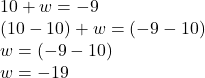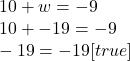## Listen i know its dumb but- solve for w 10 + w = -9

Question

Listen i know its dumb but-
solve for w
10 + w = -9

in progress 0
3 months 2021-08-21T23:18:22+00:00 1 Answers 5 views 0

w = -19

Explanation:

To solve this equation, subtract ten from both sides. This will isolate the variable (w) and allow us to find its value.If you would like to double check that the variable (w) does in fact equal negative nineteen, substitute the value of the variable in the equation and solve.Therefore, the value of w in the given equation is -19.